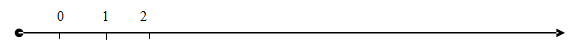#### Types of Variables

Types of Variables

Quantitative variables: variables which can be measured numerically. Examples is Number of books, process of houses and number of students.

Quantitative variables can be classified as shown below

a. Discrete Variables are variables whose values are countable

Discrete variables take non-negative and no decimal values. To get the value of this variable, we normally use counting number or whole number i.e. 0,1,2,….b. Continuous Variables are variables that can assume all numbers within a certain interval or intervals. Examples is the height of the students. We can assume any values from 1 to 7 ft, including decimals.Qualitative variables are variables which cannot be measured numerically. Examples: colour of cars, types of food and students’ grade for Semester 1.

Email based statistics assignment help - homework help at TutorsGlobe

Are you searching statistics tutor for help with Types of Variables questions?  Types of Variables topic is not easier to learn without external help?  We at www.tutorsglobe.com offer finest service of statistics assignment help and statistics homework help. Live tutors are available for 24x7 hours helping students in their Types of Variables related problems. We provide step by step Types of Variables question's answers with 100% plagiarism free content. We prepare quality content and notes for Types of Variables topic under statistics theory and study material. These are avail for subscribed users and they can get advantages anytime.

Why TutorsGlobe for assignment help

• Higher degree holder and experienced tutors network
• Punctuality and responsibility of work
• Quality solution with 100% plagiarism free answers
• Time on Delivery
• Privacy of information and details
• Excellence in solving accounting questions in excels and word format.
• Best tutoring assistance 24x7 hours